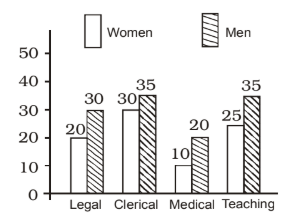## Introduction to Data Interpretation

#### Data Interpretation

1. Given below is a graph which shows the different occupations of men and women. The occupation that has larger proportion of women compared to the other three jobs is1. As per the given bar graph , we have

 Legal = 20 = 2 = 0.67 30 3

 Clerical = 30 = 0.86 35

##### Correct Option: A

As per the given bar graph , we have

 Legal = 20 = 2 = 0.67 30 3

 Clerical = 30 = 0.86 35

 Medical = 10 = 1 = 0.5 20 2

Hence , required answer is Clerical .

Direction: The follow- ing diagram shows the percentage of population of Hindus, Sikhs and Muslims with respect to total population in a town during 2005 to 2008. Study the diagram and answer the questions :1. If the total number of Hindus in 2008 was 12 lakh, the number of Muslims in 2008 was (in lakh)

1. According to given bar graph , we have
Given , Total number of Hindus in 2008 = 12 lakh
Percentage of population of Hindus in 2008 = 15%
Percentage of population of Muslims in 2008 = 20%
∴ 15% = 12 lakh

 ⇒ 1% = 12 15

##### Correct Option: B

According to given bar graph , we have
Given , Total number of Hindus in 2008 = 12 lakh
Percentage of population of Hindus in 2008 = 15%
Percentage of population of Muslims in 2008 = 20%
∴ 15% = 12 lakh

 ⇒ 1% = 12 15

 ⇒ 20% = 12 × 20 = 16 lakhs 15

Hence , Number of Muslims = 16 lakhs

1. Difference of percentage of population of Hindus in 2005 and 2008 is

1. According to given bar graph , we have
Percentage of population of Hindus in 2005 = 30%
Percentage of population of Hindus in 2008 = 15%
Required difference = Percentage of population of Hindus in 2005 - Percentage of population of Hindus in 2008

##### Correct Option: B

According to given bar graph , we have
Percentage of population of Hindus in 2005 = 30%
Percentage of population of Hindus in 2008 = 15%
Required difference = Percentage of population of Hindus in 2005 - Percentage of population of Hindus in 2008
∴ Required difference = 30 - 15 = 15%

1. Percentage decrease in Hindu population from 2005 to 2008 is

1. According to given bar graph , we have
Percent of Hindus in 2005 = 30%
Percent of Hindus in 2008 = 15%
Decrease = 30 - 15 = 15%

 Percentage decrease = Decrease × 100 Percent of Hindus in 2005

##### Correct Option: A

According to given bar graph , we have
Percent of Hindus in 2005 = 30%
Percent of Hindus in 2008 = 15%
Decrease = 30 - 15 = 15%

 Percentage decrease = Decrease × 100 Percent of Hindus in 2005

 Percentage decrease = 15 × 100 = 50% 30

1. If the total population in 2007 was 80 lakh, then the number of Hindus in 2007 was (in lakh)

1. On the basis of given graph in question ,
The total population in 2007 = 80 lakh
Percent of Hindus in 2007 = 25%
Number of Hindus = 25% of 80 lakh

##### Correct Option: D

On the basis of given graph in question ,
The total population in 2007 = 80 lakh
Percent of Hindus in 2007 = 25%
Number of Hindus = 25% of 80 lakh

 Number of Hindus = 80 × 25 = 20 lakhs 100